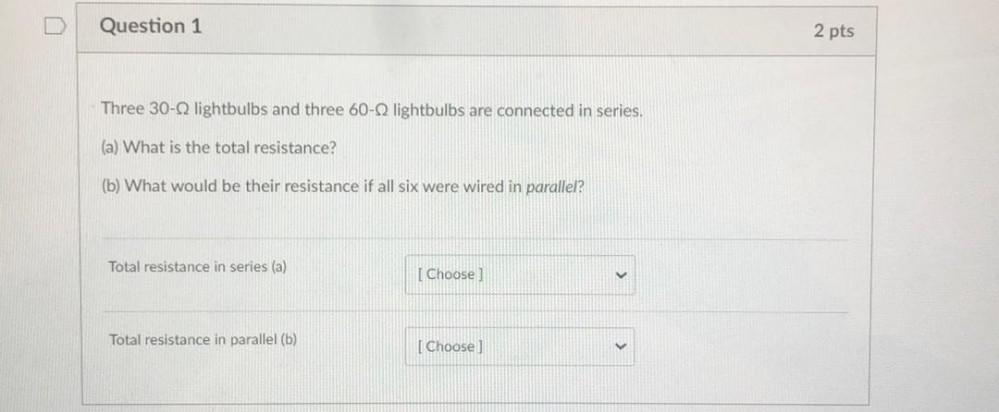Question:

# U Question 1 2 pts Three 30-Q lightbulbs and three 60-Q lightbulbs are connected in series. (a) What is the total resistance? (bU Question 1 2 pts Three 30-Q lightbulbs and three 60-Q lightbulbs are connected in series. (a) What is the total resistance? (b) What would be their resistance if all six were wired in parallel? Total resistance in series (a) [ Choose Total resistance in parallel (b) Choose]